# SAT Math : How to order decimals from least to greatest or from greatest to least

## Example Questions

← Previous 1 3 4

### Example Question #1 : How To Order Decimals From Least To Greatest Or From Greatest To Least

Order the following decimals in order from least to greatest:

2.1, 6.9, 4.8, 5.2, 8.5

6.9, 4.8, 8.5, 5.2, 2.1

2.1, 5.2, 8.5, 4.8, 6.9

8.5, 6.9, 5.2, 4.8, 2.1

2.1, 4.8, 5.2, 6.9, 8.5

2.1, 4.8, 5.2, 6.9, 8.5

Explanation:

Look at the ones place first, ignoring the decimal. The order from greatest to least is 2, 4, 5, 6, and 8. Because none of the numbers in the ones place are the same, it doesn't matter what the decimal will be on these numbers- the order will remain the same. The order is 2.1, 4.8, 5.2, 6.9, 8.5.

### Example Question #2 : How To Order Decimals From Least To Greatest Or From Greatest To Least

In the number 5,783.2935, what is the digit in the hundredths place?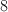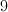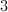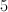Explanation:

The digit in the hundredths place is the one two decimal places to the right of the decimal. That digit is 9. Do not confuse the hundredths with the hundreds place, which is three spaces to the left of the decimal.

### Example Question #3 : How To Order Decimals From Least To Greatest Or From Greatest To Least

Which of these decimals are the greatest?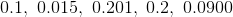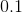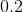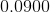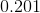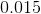Explanation:

look at the first decimal place; 0.2 and 0.201 are the largest.

0.2 = 0.200 and 0.201 > 0.200

0.201 is the greatest given decimal.

### Example Question #2 : How To Order Decimals From Least To Greatest Or From Greatest To Least

Which of the following is the greatest in value?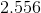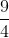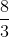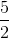Explanation:

Turn the fractions into decimals to easily compare them. You can do this quickly on your calculator.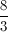is approximately 2.667, so this is the largest number.

### Example Question #5 : How To Order Decimals From Least To Greatest Or From Greatest To Least

Which of these decimals has the least value?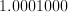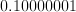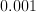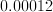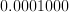Explanation:

Trailing zeros at the end of a decimal do not increase or decrease the value of a given decimal. However, zeros that appear after the decimal point and precede a non-zero number decrease the value of the number.

### Example Question #1 : How To Order Decimals From Least To Greatest Or From Greatest To Least

Put the following in order of least to greatest: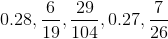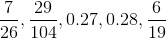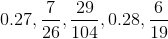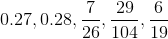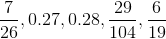Explanation:

Begin by converting each fraction into a decimal: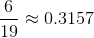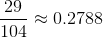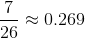Now we can put the decimals in order:

0.269, 0.27, 0.2788, 0.28, 0.3157

or: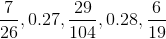### Example Question #6 : How To Order Decimals From Least To Greatest Or From Greatest To Least

Order the decimals from least to greatest.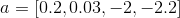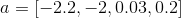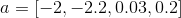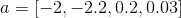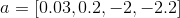Explanation:

The higher negative numbers will be the least numbers.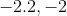The smaller of the two positive decimals is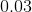.

Order from least to greatest.

The answer is:### Example Question #7 : How To Order Decimals From Least To Greatest Or From Greatest To Least

Which decimal is the greatest?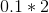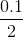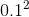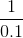Explanation:

When evaluating, we look at each choice.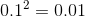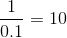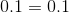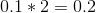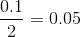It seems like when dividing with a decimal, our value is greater. Thereforeis our answer.

### Example Question #8 : How To Order Decimals From Least To Greatest Or From Greatest To Least

Which is the smallest?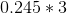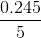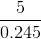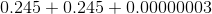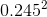Explanation:

When evaluating, we look at each choice.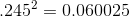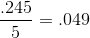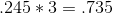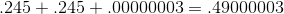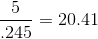Our answer is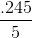being the smallest.

### Example Question #9 : How To Order Decimals From Least To Greatest Or From Greatest To Least

Ifis from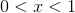which is the greatest?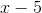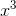The numbers are fromto. This means the values are decimals and will be small if raised to any power but greater when multiplied or added.
Ex: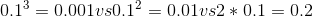Our answer is going to be.